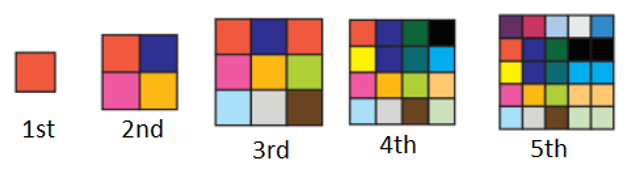Mathematics
Easy

Question

# Akira made a pattern using square tiles.If the pattern continues, the number of square tiles required to make the 7th shape is

## 30    36    45    49Hint:

## The correct answer is: 49

### We are given 5 shapes with increasing number of tiles.If we see, there is a relation between the number of shape and number of tiles. The number of tiles is the square of the number of shape.Therefore, the rule used is “the square of number of shapes"The first shape has 12 = 1 tile.The second shape has 22 = 4  tiles.The third shape has 32 = 9 tiles.The fourth shape has 42 = 16 tiles.The fifth shape has 52 = 25 tiles.We are asked to find the number of tiles in  7th shape.Seventh shape will have 72 = 49 tiles.The number of tiles in 7th shape is 49.

While solving the question, we have to be careful about the order of the sequence.

### Related Questions to study#### With Turito Foundation.#### Get an Expert Advice From Turito.